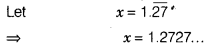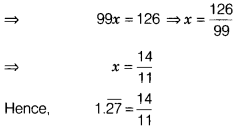# Express 1.27 as a vulgar fraction

Express 1.27 as a vulgar fraction.On multiplying both sides by 100 in Eq. (i),we get
100x= 100 × 1.2727… (1/2)
=> 100x= 127.2727…
100x = 126+ 1.2727…
100x = 126 + x [from Eq. (i)]